# The PMSU Spectroscopic Survey of Nearby M dwarfs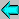PMSU 3 --- | --- INR home page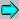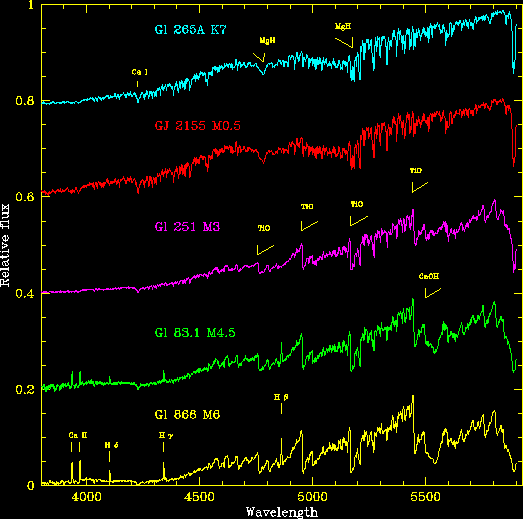# PMSU4: The nearby star luminosity and mass functions: Combining the Hipparcos and PMSU datasets

## 1. Hipparcos and the upper main sequence - a volume-limited sample

The ESA Hipparcos satellite obtained milli-arcsecond accuracy astrometry for over 118,000 stars over the whole sky. The satellite carried out a pointed survey, targeting individual objects from an input catalogue, compiled in the early 1980s based on proposals made by the European astronoical community. While that catalogue includes a few stars (and 3C273) fainter than V=12, the overwhelming majority of stars are brighter than 9th magnitude. The formal completeness limit is latitude dependent

V = 7.9 + 1.1 sin|b|
but, since the input catalogue included a series of proposals which specifically targeted known and suspected nearby stars, that sample is likely to be more complete. Jahreiss & Wielen (1997) estimate that the catalogue is effectively complete for stars brighter than MV=8.5 within 25 partsecs of the Sun. Consequently, the Hipparcos dataset almost exactly complements the PMSU survey, and allows us to extend coverage to brighter, higher-mass stars in the Solar Neighbourhood.

There are a total of 1477 stars in the Hipparcos catalogue with parallaxes larger than 40 mas, consistent with distances of less than 25 parsecs. As Figure P.8 shows, besides upper main-sequence and evolved stars, that sample includes early- and mid-type M dwarfs and white dwarfs, together a number of stars lying at somewhat unusual locations in the HR diagram. Most of the latter stars have parallax measurements with large formal undertainties, usually due to the presence of a close (physical or line-of-sight) companion. Eliminating those stars from the sample (only a couple are formally brighter than MV=8.0) leaves 844 stars. That sample includes red giants and metal-poor subdwarfs, as well as separate measurements for components in wide binary systems. Since our aim is a derivation of the luminosity function for main-sequence stars and systems including main-sequence components, the sample needs to be trimmed of those unwanted contributors.

The resulting datasets are listed at the foot of this page: there are 41 evolved stars (where "evolved" is defined based on the location on the HR diagram, see Figure P.8); 4 subdwarfs (HD 25329, HD 103095, HD 120559 and HD 145417, with -0.9 < [Fe/H] < -1.6); and measurements of 35 secondary components. We have also searched the literature to identify binary/multiple systems and determine, as far as possible, their apparent and absolute magnitudes. Figure P.8 plots the (MV, (B-V)) colour-magnitude diagram outlined by the remaining 764 primaries or single stars. Where necessary, the magnitudes listed by Hipparcos have been adjusted to allow for the contribution of unresolved secondaries, so a few stars slipe below the nominal MV=8.0 limit. As discussed below, several of those stars are not in the PMSU sample.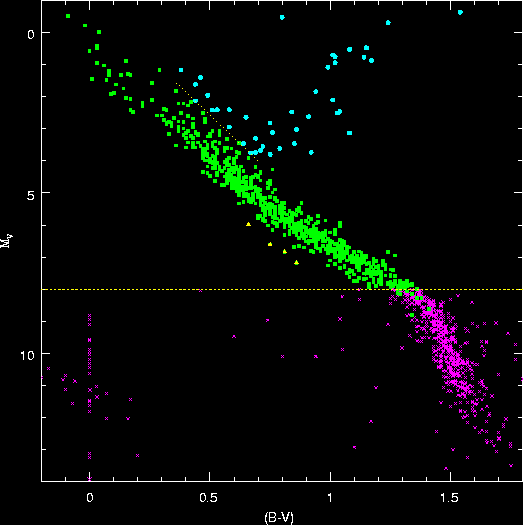Figure P.8: (MV, (B-V)) colour-magnitude diagram for stars in the Hipparcos catalogue with pi > 40 mas. Solid green squares mark main-sequence stars in the 25-parsec sample (adjusting the magnitudes for stars in binaries); yellow points are halo subdwarfs; cyan dots are evolved stars.

Having trimmed the sample to main-sequence stars only, we can test Jahreiss & Wielen's conjecture that the Hipparcos catalogue is complete to 25 parsecs for stars with MV < 8.5. Figure P.9 plots the average density as a function of distance for systems including main-sequence stars with absolute magnitudes in the range 4 < MV < 8. The innermost point plots the average density for d < 16 pc.; subsequent points plot the density in spherical shells from 16 to 18, 18 to 20, 20 to 22 and 22 to 25 parsecs. There is no evidence for a significant decline in densities in the outermost shell, even for the faintest stars.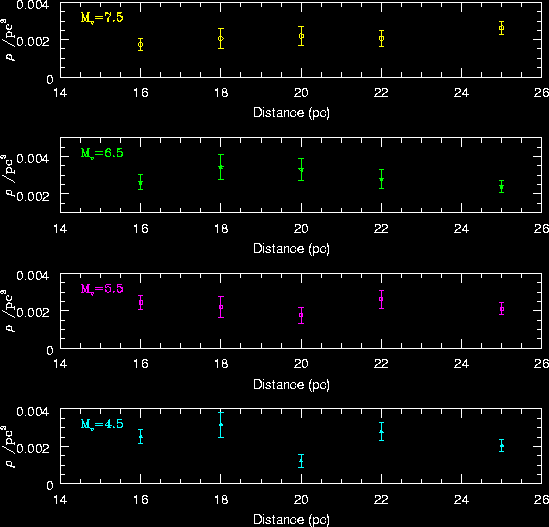Figure P.9: The run of number density with distance for Hipparcos main-sequence stars with 4 < MV < 8.

## 2. Multiplicity

The 25-parsec upper main-sequence sample includes 764 systems: 538 single stars, 204 binaries (including 8 low-amplitude spectroscopic binaries from the Keck/Lick survey, summarised by Nidever sl et al., 2002), 22 triples and 4 quadruple systems. The resultant multiplicity fraction is only 30.1+/-2.4 %, somewhat lower than the 44 % derived by Duquennoy & Mayor (1991; DM91) in what has become the standard reference for multiplicity amongst solar-type stars. This could reflect incompleteness in surveying the Hipparcos sample - and there are at least 14 % of the sample which lack any radial velocity measurements - but it might also reflect the fact that the sample extends to fainter, lower-mass stars than the DM91 survey. M dwarfs have a lower multiplicity, between 30 and 35% - see Fischer & Marcy, 1992; Reid & Gizis, 1997). Finally, there is a possibility that the DM91 sample could be biased. The intention was to pick a volume-limited sample, but since the stars were selected from the CNS2, the parallax measurements were subject to the systematics illustrated in Figure P.5 . Since all of the stars were observed by Hipparcos, we can check whether such a bias exists.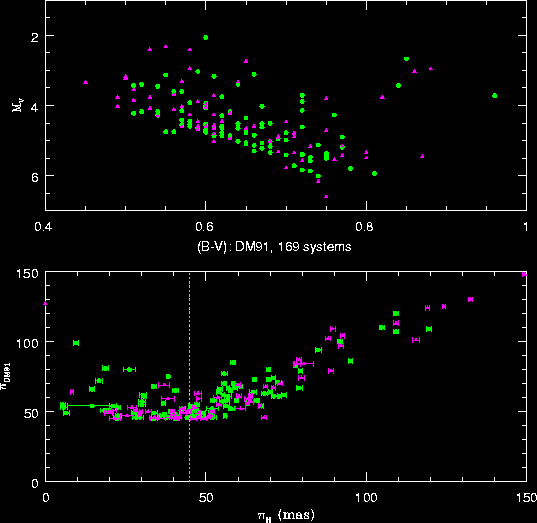Figure P.10: The DM91 sample. The lower panel compares the CNS2 and Hipparcos parallax measurements, with the nominal distance limit of 45 mas outlined. The upper panel plots the distribution in the HR diagram. Magenta points are single stars, green points are known binaries.

The DM91 sample includes stars with spectral types between F7 and G9, parallaxes exceeding 45 mas and declinations above -15o. Figure P.10 compares the CNS2 and Hipparcos parallax measurements for the 169 systems from DM91 which meet those criteria. Only 102 actually lie within the formal distance limit. However, 42 of those 102 systems are double or multiple, so the multiplicity fraction is 41+/-7 %, statistically identical with DM91's calculation (and only 1.5 sigma above the Hipparcos 25-parsec binary fraction). Thus observational bias is not a likely source of the difference between the DM91 and Hipparcos datasets.

Given this consistency check, we can use DM91's analysis of their sample to estimate a likely upper limit to the binary fraction in the Hipparcos sample. Low-amplitude spectroscopic binaries, either wide, long-period systems, high inclination systems, or high mass-ratio systems, are more difficult to detect. Given the available statistics, DM91 calculated that their observations underestimated the true binary fraction by about one third, deriving a total multiplicity of 57 %. In passing, we note that 47 of the 60 "single" stars from the revised DM91 sample are on the Keck/Lick program; only one has a velocity r.m.s. exceeding 100 m/sec. Nonetheless, this value provides a reasonable upper limit to the likely contribution of binaries to the Hipparcos sample - so we have allowed for that possibility by giving double weight to each of the known binary companions.

## 3. The luminosity function

Our goal is constructing a luminosity function - the number of stars/systems per unit absolute magnitude per unit volume. The statistics are listed in the following table, combining both the Hipparcos 25-parsec and PMSU datasets. The distance limits for companions to Hipparcos 25-parsecs primaries are based onMV (companion), and match those adopted for the PMSU sample.

Table P4: The luminosity function for nearby stars
MV Hipparcos PMSU4 Phisys Phistars
Nsys Nsec(1) Nsec(2) Nsys Nsec(1) Nsec(2) Nsupp systems pc-3 x 104 stars pc-3 x 104
-0.5 3 0.46 0.46
0.5 5 1 1 0.76 0.92
1.5 15 2 2 2.29 2.60
2.5 36 1 1 5.50 5.65
3.5 79 9 9 12.07 13.45
4.5 151 15 15 23.07 25.36
5.5 147 19 19 22.46 25.36
6.5 181 32 32 27.65 32.54
7.5 143 24 24 21.85 25.52
8.5 8 41 27 103 7 7 6 32.01 40.16
9.5 23 13 92 7 5 3 50.21 58.26
10.5 28 4 64 15 9 74.24 88.16
11.5 21 2 66 17 11 3 76.56 91.06
12.5 15 6 71 25 17 1 82.36 107.30
13.5 7 23 14 6 73.21 92.31
14.5 13 6 3 41.38 50.93
15.5 5 1 4 12 5 101.86 248.28
16.5 1 2 2 25.46 76.39
17.5 2 6 1 50.93 76.39

Column 2 lists the number-magnitude distribution of single stars and primary stars in the Hipparcos 25-parsec sample; column 3 gives the magnitude distribution for known companions; column 4 lists the companion star distribution after applying the absolute magnitude-dependent distance limits given in Table 1. Columns 5, 6 and 7 provide the same statistics for the PMSU4 sample, and column 8 gives the contribution from the supplementary stars listed in Table 3. Combining the samples, the luminosity function due to primaries and single stars is listed in column 9, while the space densities given in column 10 include the contribution from the companions listed in columns 4 and 7.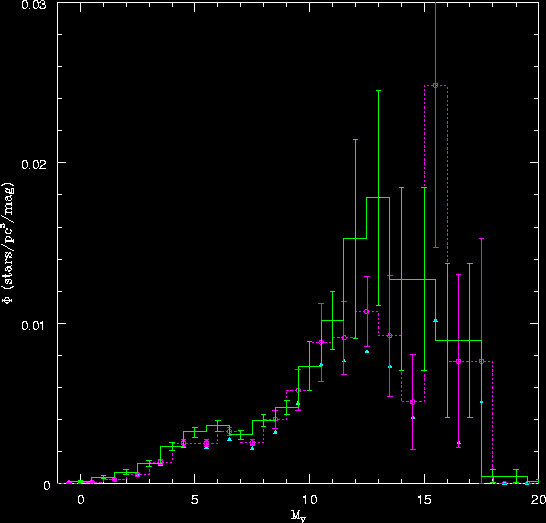Figure P.11: The nearby-star luminosity function: the green histogram plots the results derived by Wielen ey al (1983); the magenta histogram plots our results, with the blue points marking the contribution from single stars and primaries (i.e., the systemic luminosity function)

Figure P.11 compares the resulting luminosity function against the results derived in 1983 by Wielen et al, using data from the CNS2 and its supplement. The current luminosity function tends to lie below the older analysis, reflecting the systematic errors in pre-Hipparcos parallax measurements, which tended to place stars nearer the Sun, and therefore inflated the local density estimates. Those effects are particularly evident amongst the M dwarfs - as discussed previously. Figure P.12 shows the effect of giving double weight to the Hipparcos binaries; the resulting function lies somewhat closer to the Wielen et al calculation at brighter magnitudes, but the overall effect is not particularly significant.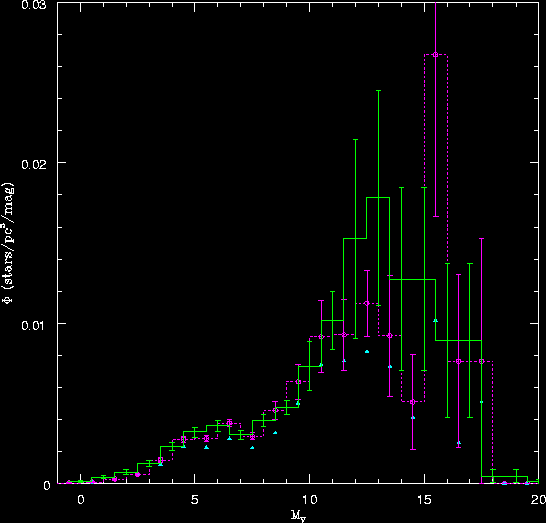Figure P.12: The nearby-star luminosity function derived when double weight is assigned to the Hipparcos 25-parsec secondaries. The symbols have the same meaning as in Figure P.11. The effect is not particularly significant.

## 4. The mass-luminosity relation

Given a main-sequence luminosity function, we need a mass-luminosity relation to transform the results to give a mass function. That relation is defined using observations of stars in eclipsing or astrometric binaries. In general, eclipsing binaries provide the calibrating stars at high masses (> 1 MSun); astrometric binaries, plus a handful of eclipsing systems (YY Gem, CM Dra, GJ 2069) provide the calibration at sub-solar masses. Henry & McCarthy (1993) undertook the first thorough analysis extending to the hydrogen-burning limit, matching the available data with a three-component fit. Since that study, data have become available for more binary systems, while continued observations have led to better orbit determinations, and better mass estimates, for other sysyems (Segresan et al, 2001). Those improved data have been analysed by Delfosse et al (2001) to derive revised (mass, absolute magnitude) relations. Figure P.13 compares their (mass, MV) relation against the Henry & McCarthy dataset, and also shows a polynomial fit to Andersen's compilation of data for higher-mass systems.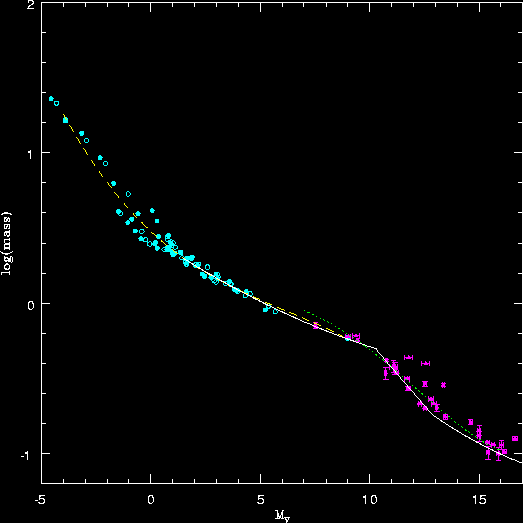Figure P.13: The mass-luminosity relation: cyan point mark data for eclipsing binaries from Andersen (1991); green points plot the revised masses for low-mass systems from the compilation by segresan et al (2001); the dotted line marks Delfosse et al's (2001) fit to the low-mass data; the dashed line is a fit to the Andersen dataset; and the solid line plots the Henry & McCarthy relation.

Kroupa, Tout & Gilmore (1993) followed a different approach to deriving a (mass, MV) relation. Rather than fit the data directly, they adopt a reference luminosity function, and choose a functional form for the mass function (a three-component power-law, with breaks at 0.5 and 1.0 solar masses). The (mass, MV) relation is then varied to give the lowest residuals with respect to the observed datapoints. As Figure P.14 shows, The resulting relation is a close match to the Delfosse et al empirical fit at masses below ~0.5 MSun, but predicts lower masses than all fo the other relations for 8 > MV > 3. It does provide a better match to the fiducial solar point.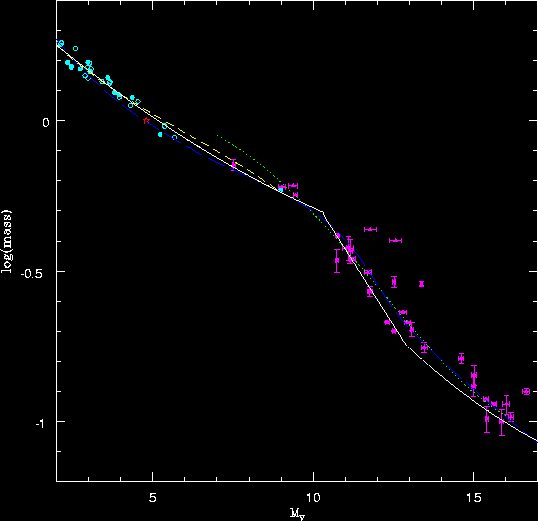Figure P.14: The mass-luminosity relation: the symbols have the same meaning as in Figure P.13, withe the addition of the blue long-dashed line marking the KTG (mass, MV) relation, and the red star marking the location of the Sun.

## 5. The present-day mass function

Figure P.15 shows the results of applying (mass, MV) relations to the nearby-star data. In each case, masses are derived on a star by star basis - i.e. we use the (mass, MV) relation to estimate a mass for each star, then combine the results to deribe the mass function. There are two composite (mass, MV) relations: the Delfosse et al fit combined with the empirical fit to the Andersen dataset, with the break between the two set at MV = 10.0; and the KTG relation combined with the Andersen fit, with the break set at MV=3.5. The former calibration is used to analyse both the straight observational dataset (i.e. the data used to derive Figure P.11) and the dataset where double weight is given to the Hipparcos 25-parsec secondaries (the data used to derive Figure P.12).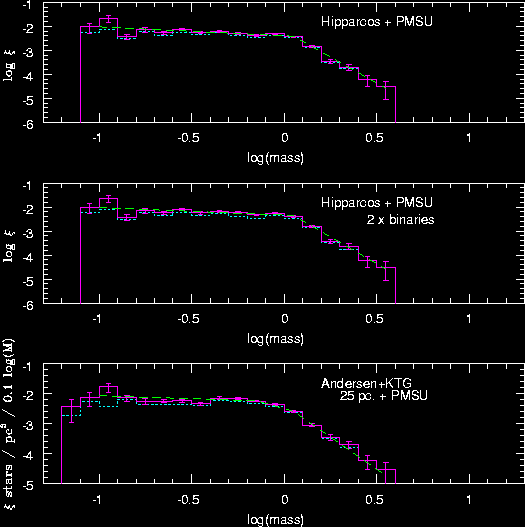Figure P.15: The present-day mass function: the uppermost panel plots the results of transforming the luminosity function plotted in Figure P.11 to masses using a composite (mass, MV) relation derived by combining the Delfosse et al fit with our polynomial fit to the Andersen dataset; the middle panel shows the effect of giving double weight to the Hipparcos binaries (i.e. applying the same conversion to the Figure P.12 data); the lowermost panel shows the mass function derived by combining the KTG and Andersen fits,

The resulting mass functions are plotted in Figure P.16, and are in generally good agreement. Fitting the results with power-law mass functions, where alpha=2.35 is the Salpeter slope, we find:

1. alpha = 1.35 for M < 1 solar mass, alpha = 5.2 for M > 1 solar mass for both analyses based on the Andersen + Delfosse et al (mass, MV calibration;
2. alpha = 1.3 for M < 0.6 solar masses, alpha = 2.8 for 0.6 to 1.0 solar masses, and alpha = 4.8 for masses above 1 solar mass, for the KTG+Andersen (mass, MV calibration.
It is not surprising that the two changes of slope in the last mass function match those used to define the (mass, MV) relation.

## 6. The initial mass function

The mass functions derived in the previous section reflect the distribution of masses amongst stars in the Solar Neighbourhood at the present time. A more fundamental parameter is the initial mass function - the distribution of masses at the time of formation. We can derive that function from the present-day mass function by applying three corrections:

1. a correction for the variation in main-sequence lifetime with stellar mass - higher-mass stars have lifetimes shorter than the age of the galactic disk, so only the most recently formed stars remain on the main sequence. In order to compensate for the evolved fracton, we need not only estimates of the main-sequence lifetimes (which can be derived from evolutionary models), but also an estimate of the star formation history of the disk. We assume a constant star formation rate and take the main-sequence lifetimes from Schaller et al (1992).
2. a correction for the distribution of stars perpendicular to the Galactic Plane - velocity dispersion increases with age, and the vertical scaleheight varioes accordingly. We have modelled the distribution as an exponential, scaleheight 250 parsecs for MV > 4; 170 parsecs for 3 < MV < 4; and 100 parsecs for the brightest stars (see Siegel et al, 2002). For this density distribution, the local surface density is simply the volume density multiplied by twice the scaleheight.
3. a correction for the local mix of populations - about 10% of local stars are members of the thick disk population, an old stellar population with a more extended vertical density distribution. We allow for their contribution to the local numberdensity of stars with MV > 4. <\ol>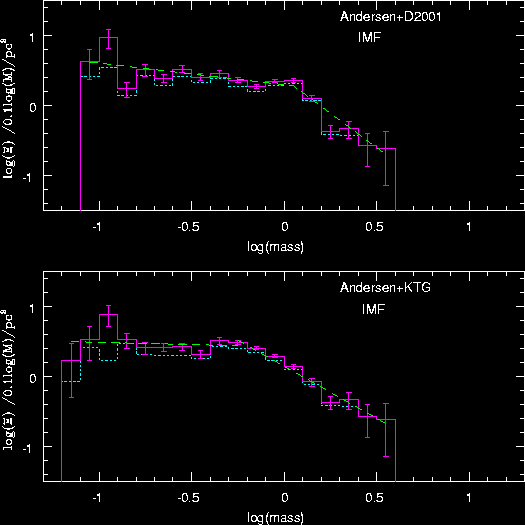Figure P.16: The initial mass function: the upper panel plots results for the Delfosse et al + Andersen (mass, MV) relation; the lower plots results for the KTG + Andersen transformation. As in Figure P.15, the dashed lines show the best-fit power-law relations.

Figure P16 shows the IMFs derived from the Andersen+Delfosse et al and Andersen+KTG present-day mass functions. The two functions are very similar in form, both well-matched by two power-law components, with the main difference being the location of the change in slope. The parameters are as follows:

1. Andersen+Delfosse et al - alpha = 1.3 for M < 1 solar mass, alpha = 2.8 at higher masses; total surface density of 41.2 solar masses / pc2;
2. Anderse+KTG - alpha = 1.1 for M < 0.6 solar mass, alpha = 2.5 at higher masses; total surface density of 40.7 solar masses / pc2;
In both cases, thick disk dwarfs contribute a further 15 solar masses / pc2 (placing 10% of local stars in the thick disk, with a scaleheight of 1 kiloparsec).

Neither of the IMFs show evidence for a change in the power-law index near the hydrogen-burning limit. As discussed in more detail by Reid {\sl et al.} (1999), direct determination of the mass function at sub-stellar masses in the field is complicated by the short visible lifetimes of brown dwarfs, and the consequent necessity of extrapolating the observed numbers to take account of systems which have cooled below the detection limit. In principle, this is the same issue affecting upper-main sequence stars; in practice, the uncertainties are more severe, since all brown dwarfs effectively follow the same cooling track in the HR diagram. As a result, it is not possible to assign a mass to an individual brown dwarf without prior knowledge of its age. Moreover, the the slower cooling time of higher-mass brown dwarfs means that they effectively dominate in any magnitude-limited sample. The current best estimate for the field, based on comparing simulations to the observed surface density of L and T dwarfs in the 2MASS and SDSS surveys, gives alpha ~ 1.3; more significantly, alpha < 2, so there is no question of hiding substantial amounts of dark matter as disk brown dwarfs (Reid et al, 1999). Observations of young clusters suggest a somewhat flatter distribution, 0.5 < alpha < 1.

## The Hipparcos 25-parsec sample

All files are ascii, and most include the following parameters:
Hipparcos number, MV. VT, BT, (B-V)T,sigmaV, sigmaB, Vlit, (B-V)lit, pi, sigmapi, muRA, mudec, sigmamu(RA), sigmamu(Dec), RA, Dec
MV is based on the literature photometry, and the astrometric parameters are in milliarcseconds. Some of the files also list a more common identifier for individual stars.
The list of companions gives
Hipparcos number of primary, V(companion), MV, distance (pc), binary type, spectral type, name and comments
Some of the apparent (hence absolute) magnitudes and spectral types are estimated rather than observed.

## References

• Andersen, J. 1991, Astr. Ast. Rev. 3, 91
• Delfosse, X., Forveille, T., Segransan, D., Beuzit, J.-L., Udry, S., Perrier, C., Mayor, M. 2000, A&A, 364, 217
• Duquennoy, A., Mayor, M., 1991, A&A, 248, 485
• Fischer, D.A., Marcy, G.W. 1992, ApJ, 396, 178
• Henry, T.J., McCarthy, D.W. 1993, ApJ, 350, 334
• Jahreiss, H., Wielen, R. 1997, Proc. ESA Stmp. 402, Hipparcos - Venice '97, ESA Publications, Noordwijk, p. 675
• Kroupa, P., Tout, C.A., Gilmore, G.F. 1993, MNRAS, 262, 545
• Kroupa, P. 2001, MNRAS,
• Meusinger, H., Schilbach, E., Souchay, J. 1996, A&A, 312, 833
• Miller, G.E., Scalo, J.M. 1979, ApJS, 41, 513
• Parenago, P.P. 1950, AZh., 27, 150
• Reid, I.N., & Gizis, J.E. 1997, AJ, 113, 2246
• Reid, I.N., Kirkpatrick, J.D., Liebert, J., Burrows, A., Gizis, J.E., Burgasser, A., Dahn, C.C., Monet, D., Cutri, R., Beichman, C.A., \& Skrutskie, M 1999, ApJ, 521, 613
• Schaller, G., Schaerer, D., Meynet, G., \& Maeder, A.\ 1992, A&AS, 96, 269
• Siegel, M.H., Majewski, S.R., Thompson, I.B., Reid, I.N. 2002, AJ, subm.
• Wielen, R., Jahreiss, H., Kruger, R., 1983, IAU Colloquium 76, ed. A.G. Davis Philip \& A.R. Upgren, p. 163 (Davis-Philip Press, Schenectady)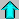NStars home pagePMSU main pageINR home page

page by Neill Reid, last updated 28/02/2002